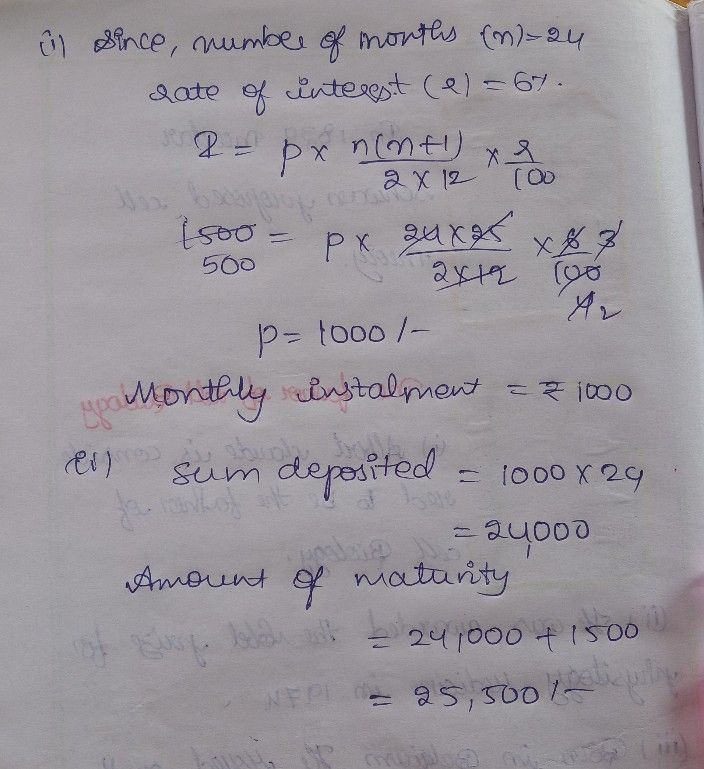Symbol
ProblemParvesh has a recurring deposit account in a bank for $2$ years at $6%p.a$ simple interest. If he gets Rs. $1500$ as interest at the time of maturity, find: $\left(1\right)$ the monthly instalment $\left(11\right)$ the amount of maturity.
10th-13th grade
Other
Search count: 106
SolutionQanda teacher - NeelimaStudent
thank you